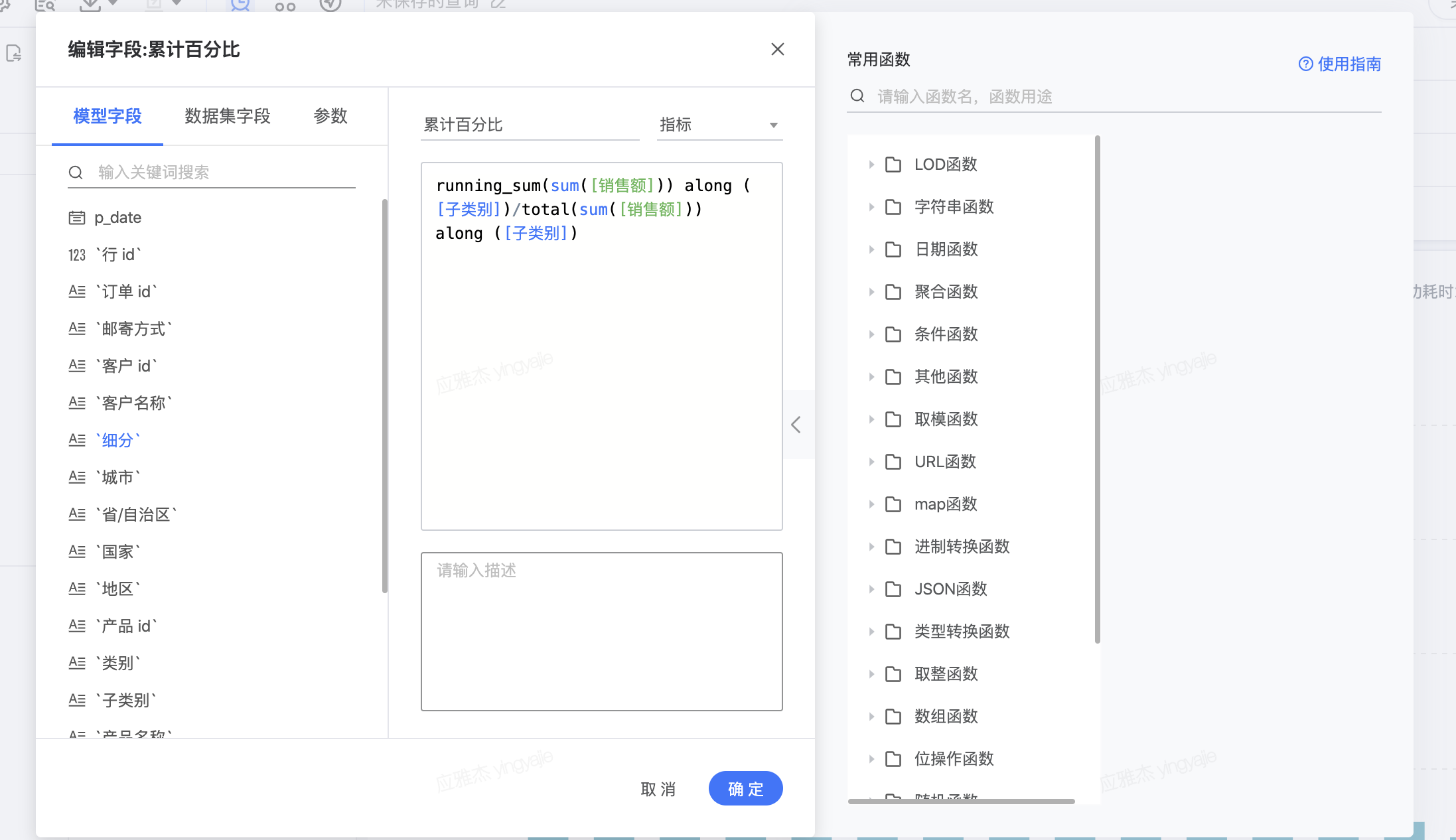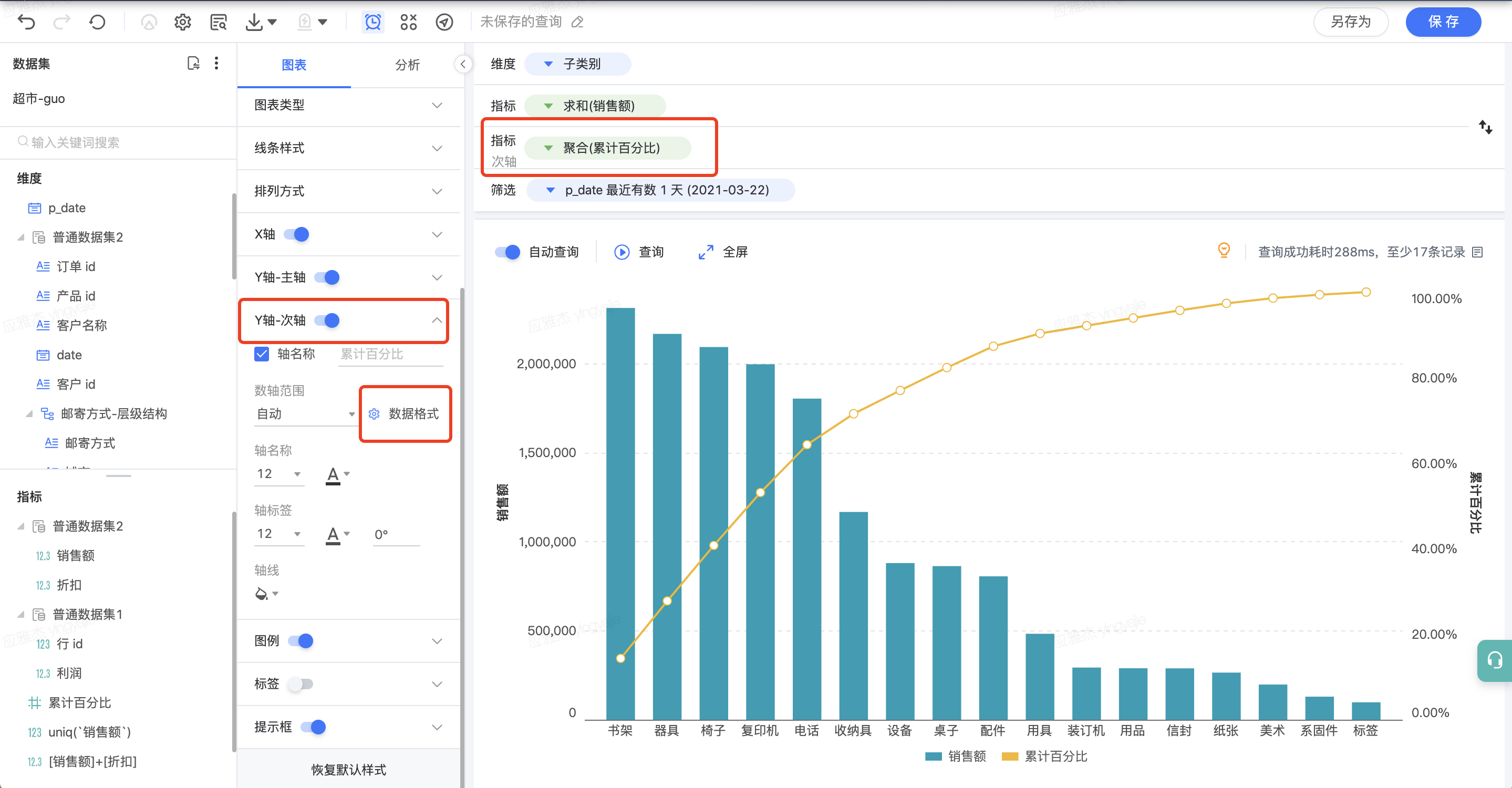You need to enable JavaScript to run this app.

# 表计算函数说明及常用场景示例

1. 使用场景

2. 常见表达式
• 表计算表达式仅能作为指标应用

• along的维度必须存在于查询面板中，否则报错

## TOTAL( <指标> ) along ( <维度>)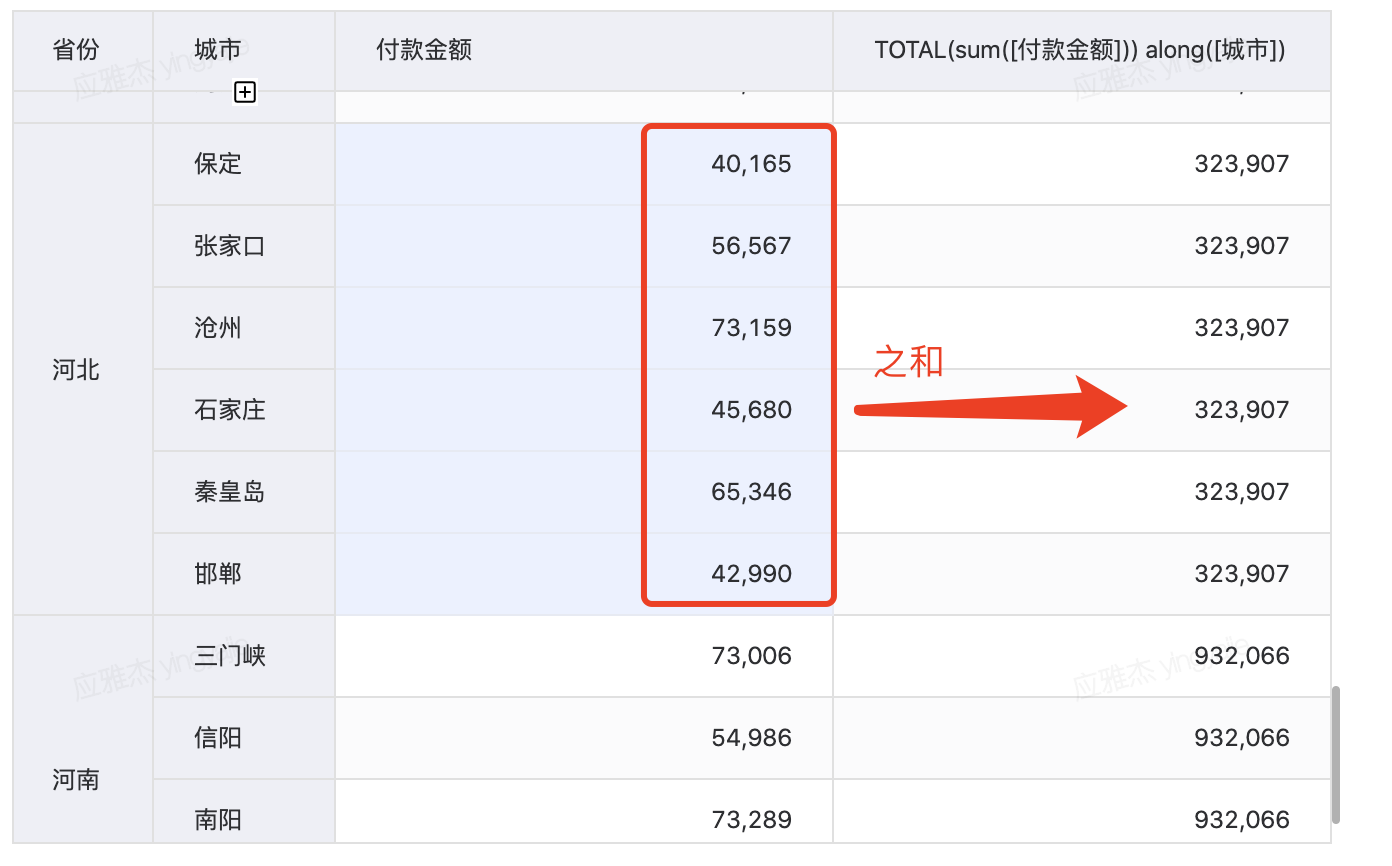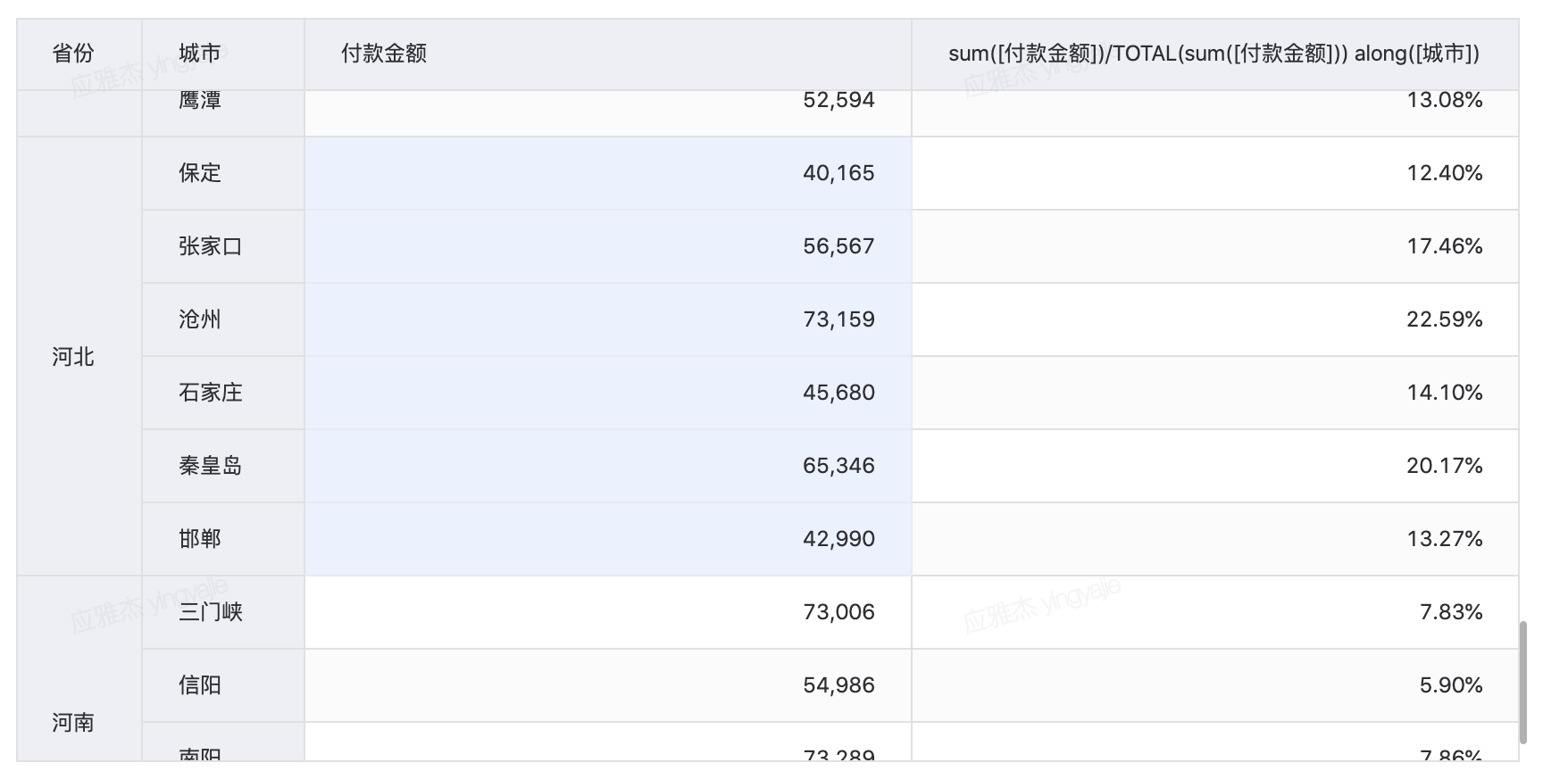## 2.1 RUNNING_SUM( <指标> )along( <维度> )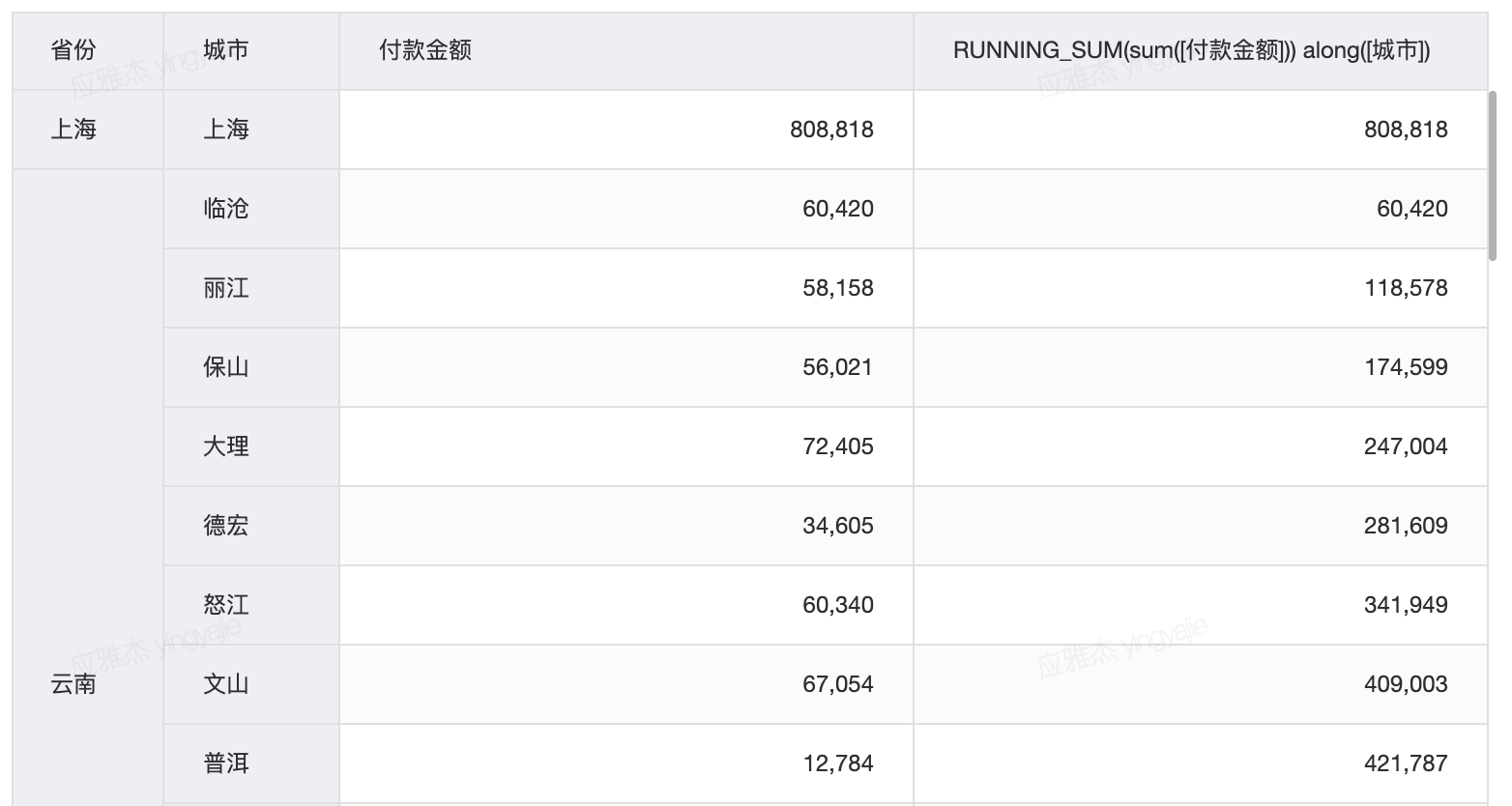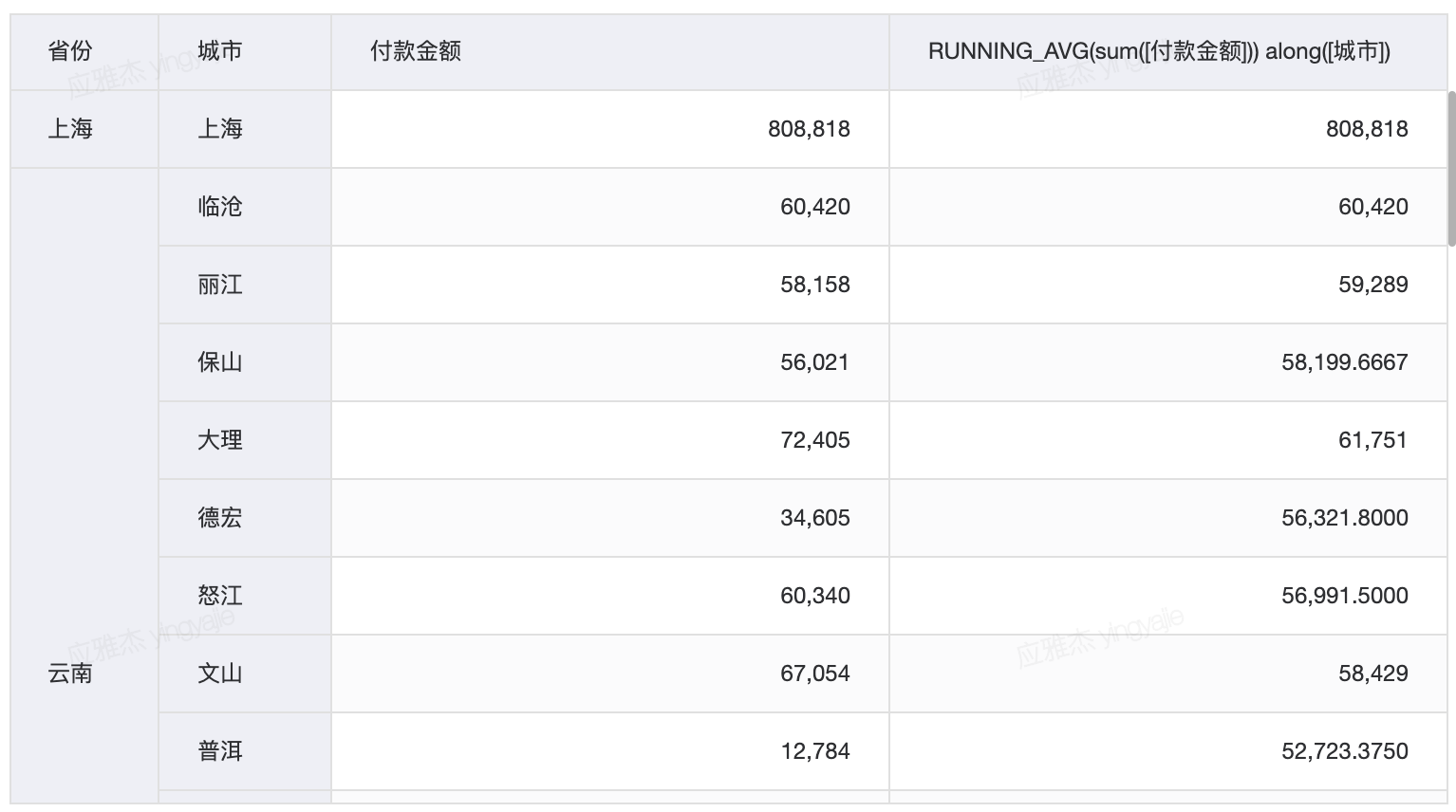## 2.2 RANK( <指标> ,'asc'/'desc') along( <维度> )

RANK(sum([利润]), “asc”) along [地区]，利用此函数，可以按照地区的利润求和进行升序排名，其中的排名有并列且会跳过并列名次，若销售额分别为(6, 7, 7, 9) 则排名结果为(4, 2, 2, 1)。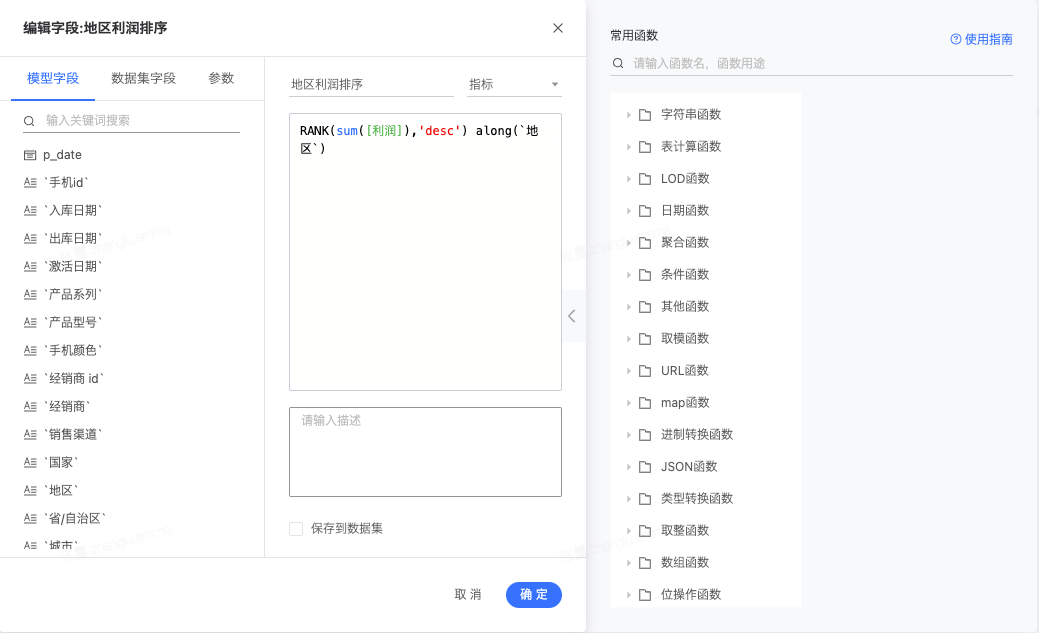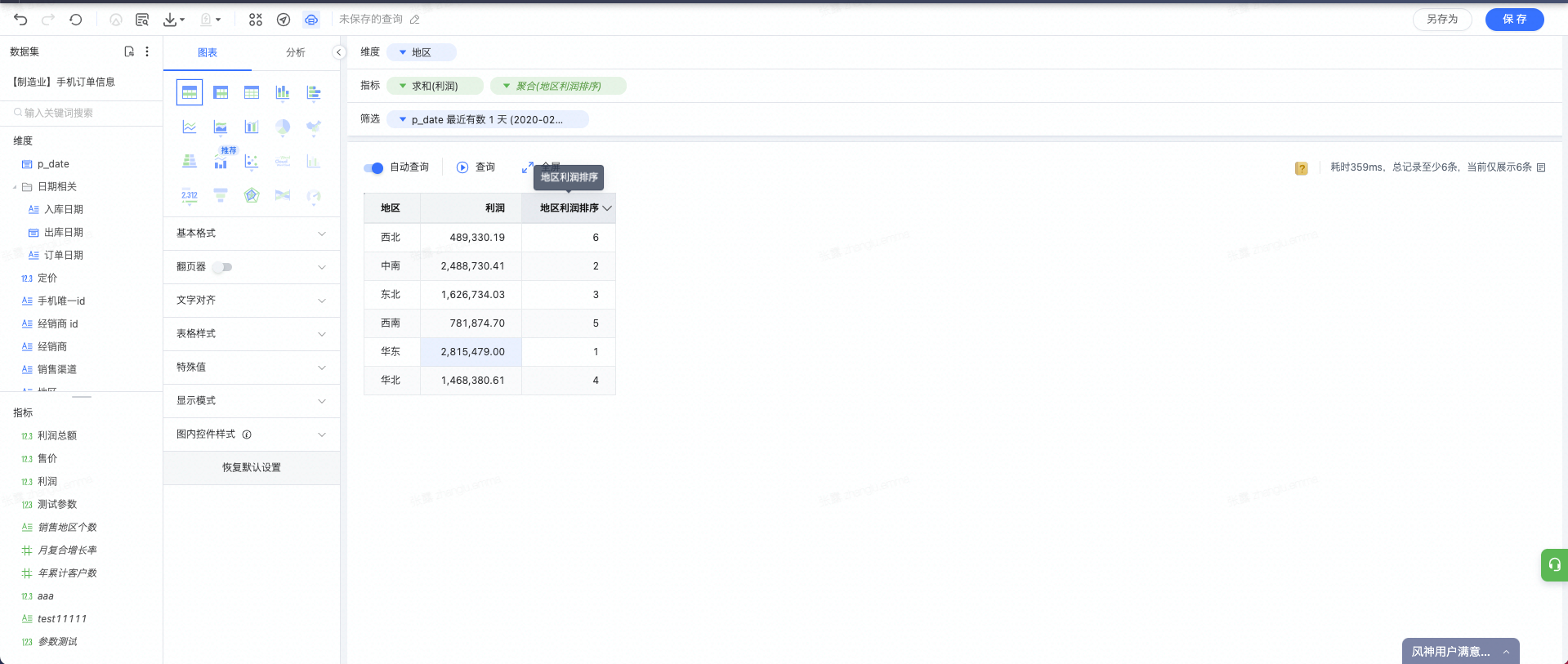## 2.3 RANK_PERCENTILE( <指标> ,'asc') along( <维度> )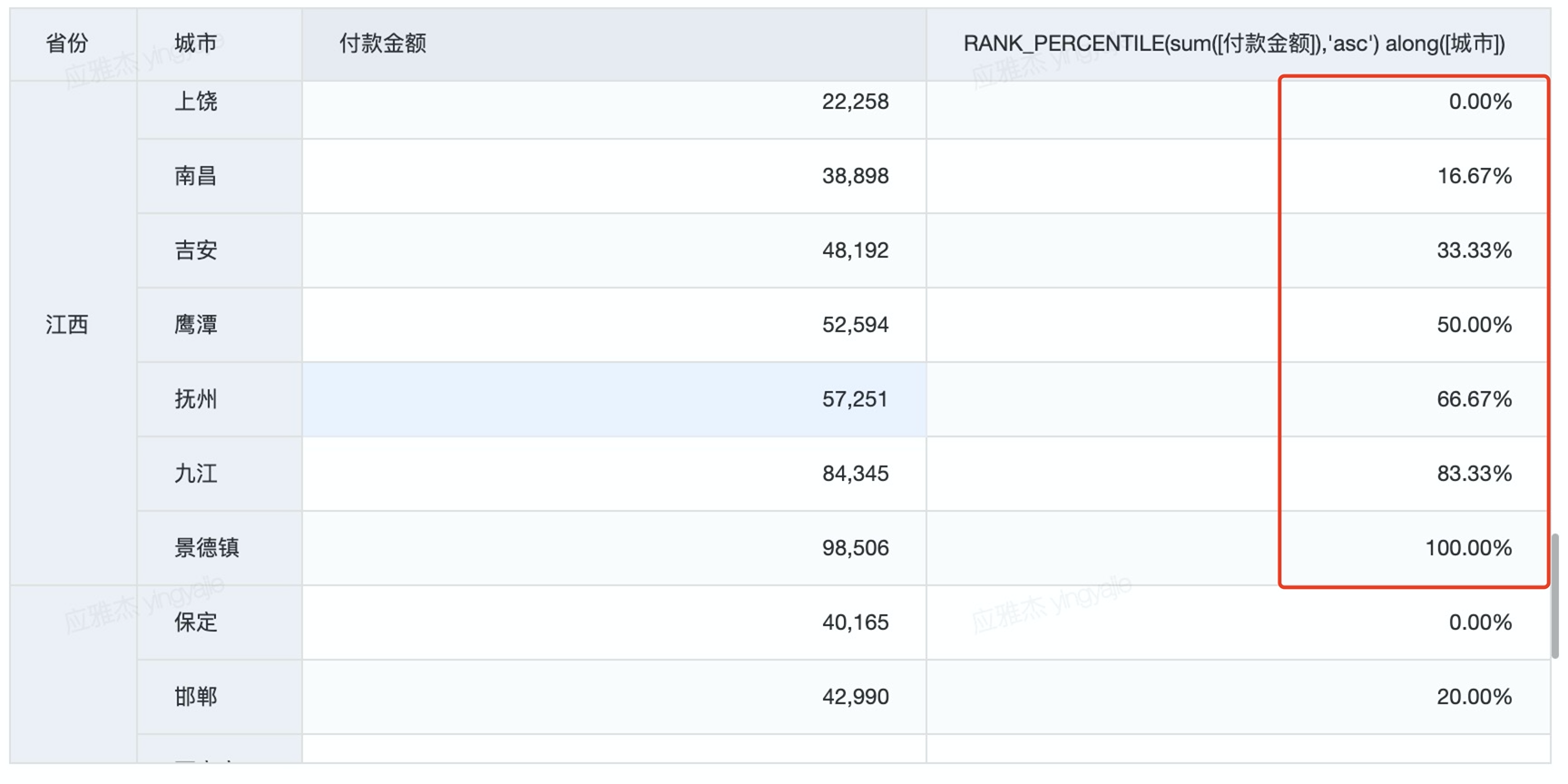## 2.4 WINDOW_AVG( <指标> ,start,end) along( <维度> )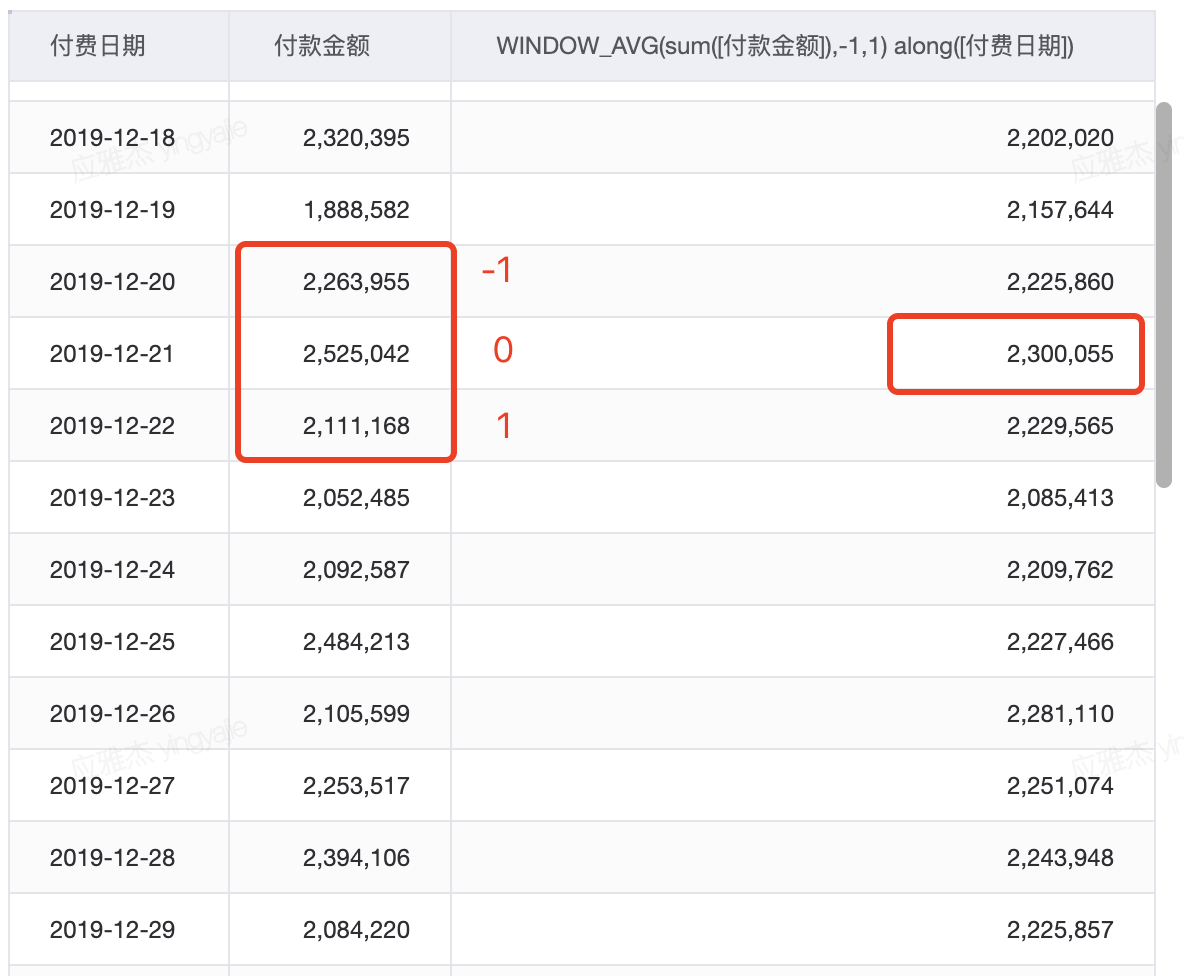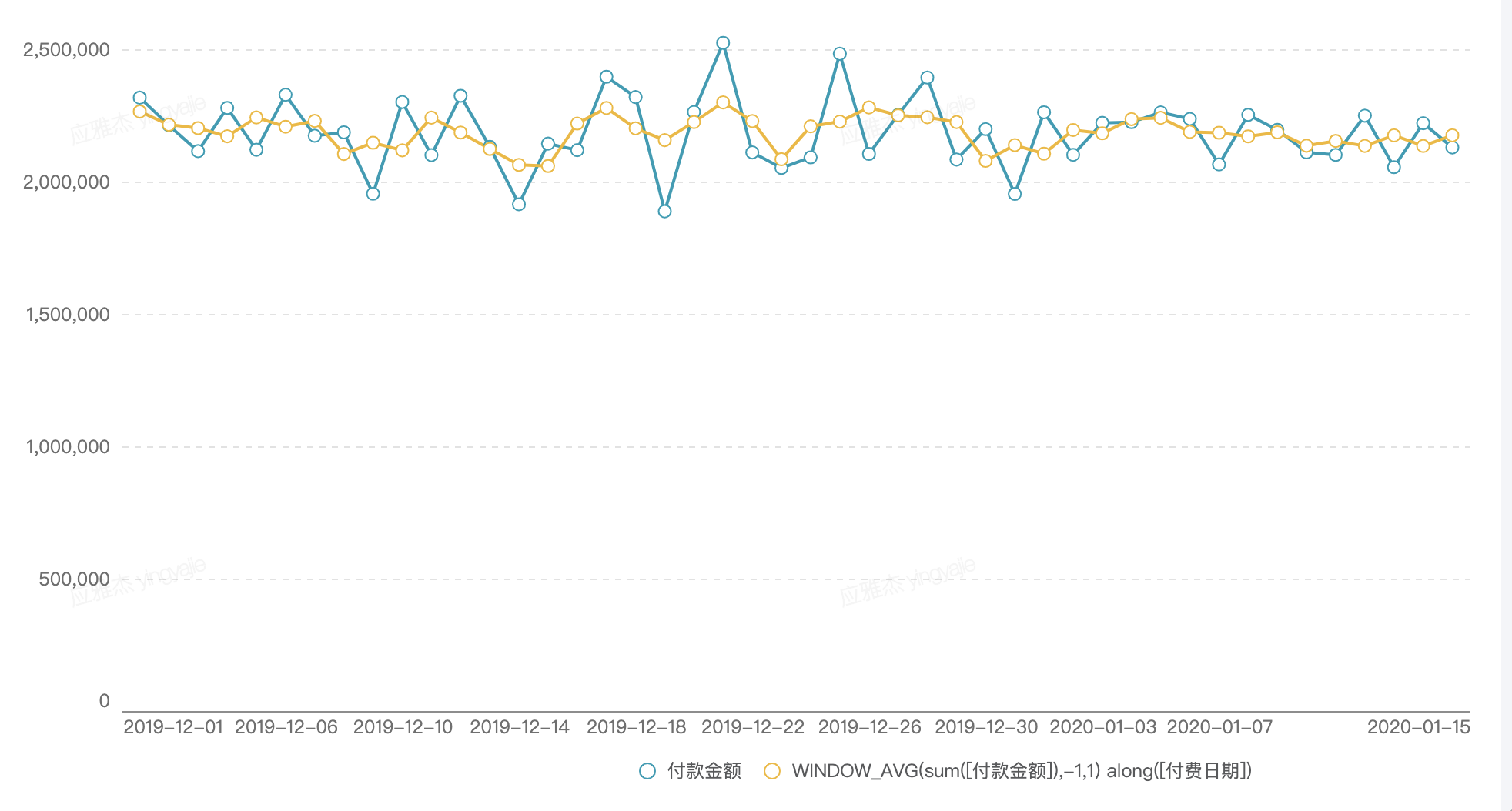## 2.5 LOOKUP( <指标> , 偏移量) along( <维度> )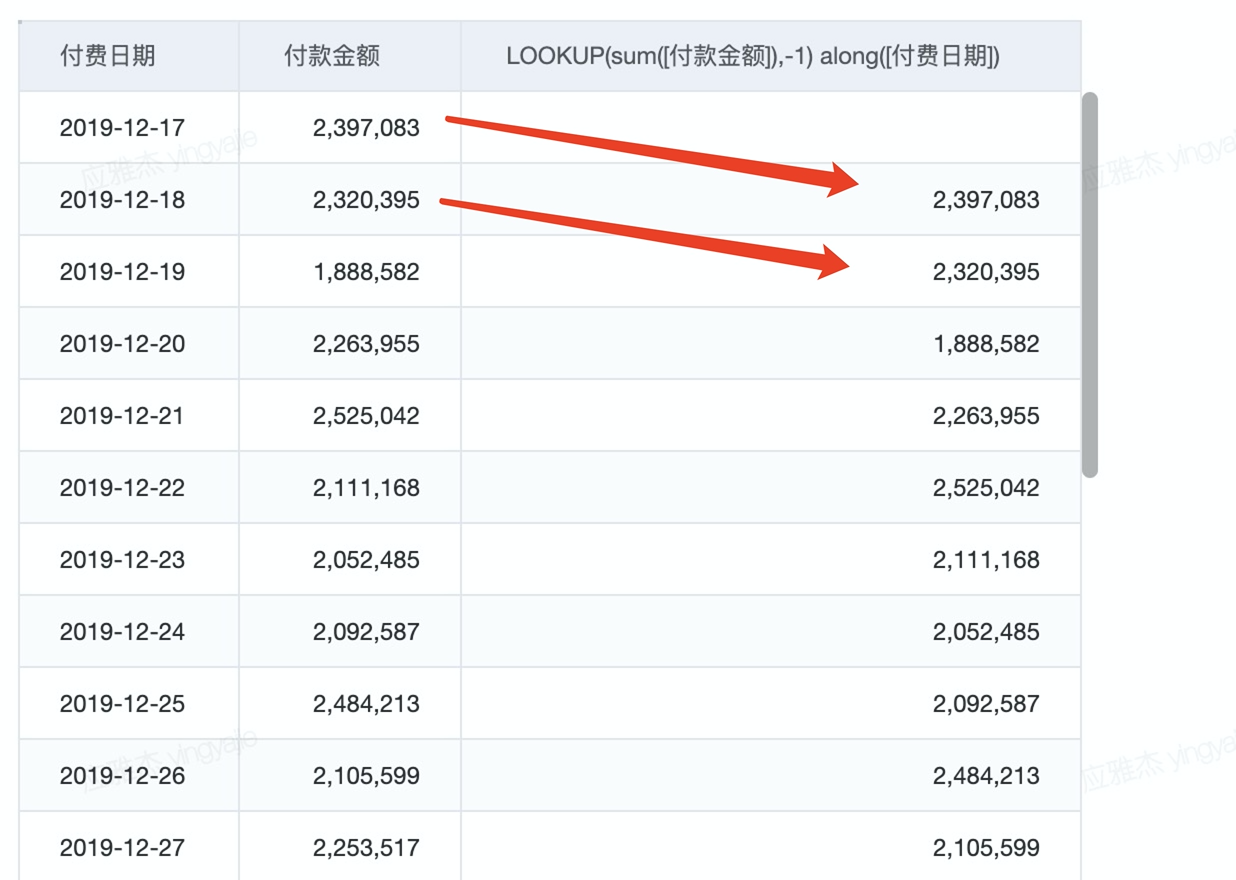3. 经典应用

## 3.1.1 示例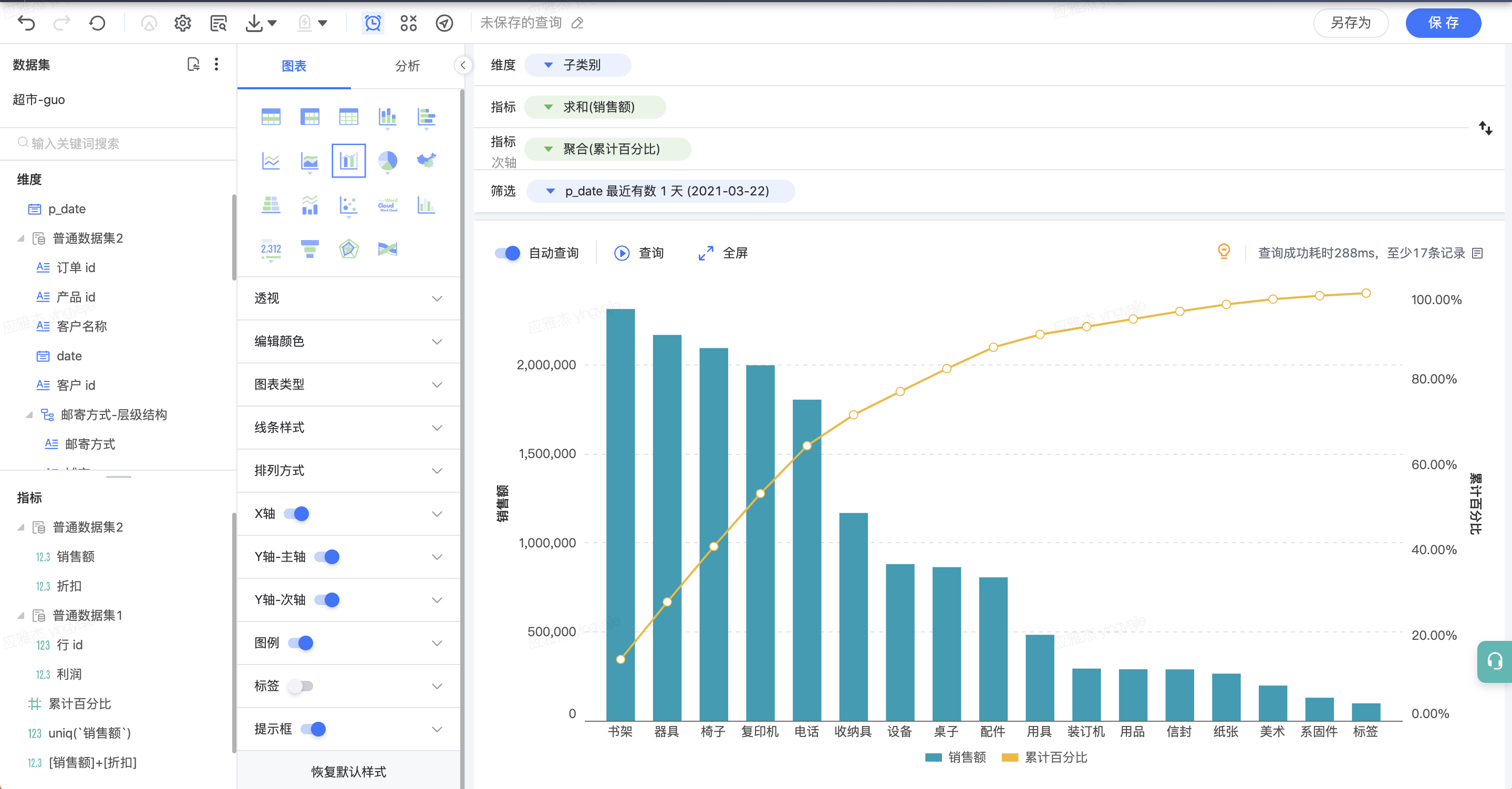### 3.1.2操作步骤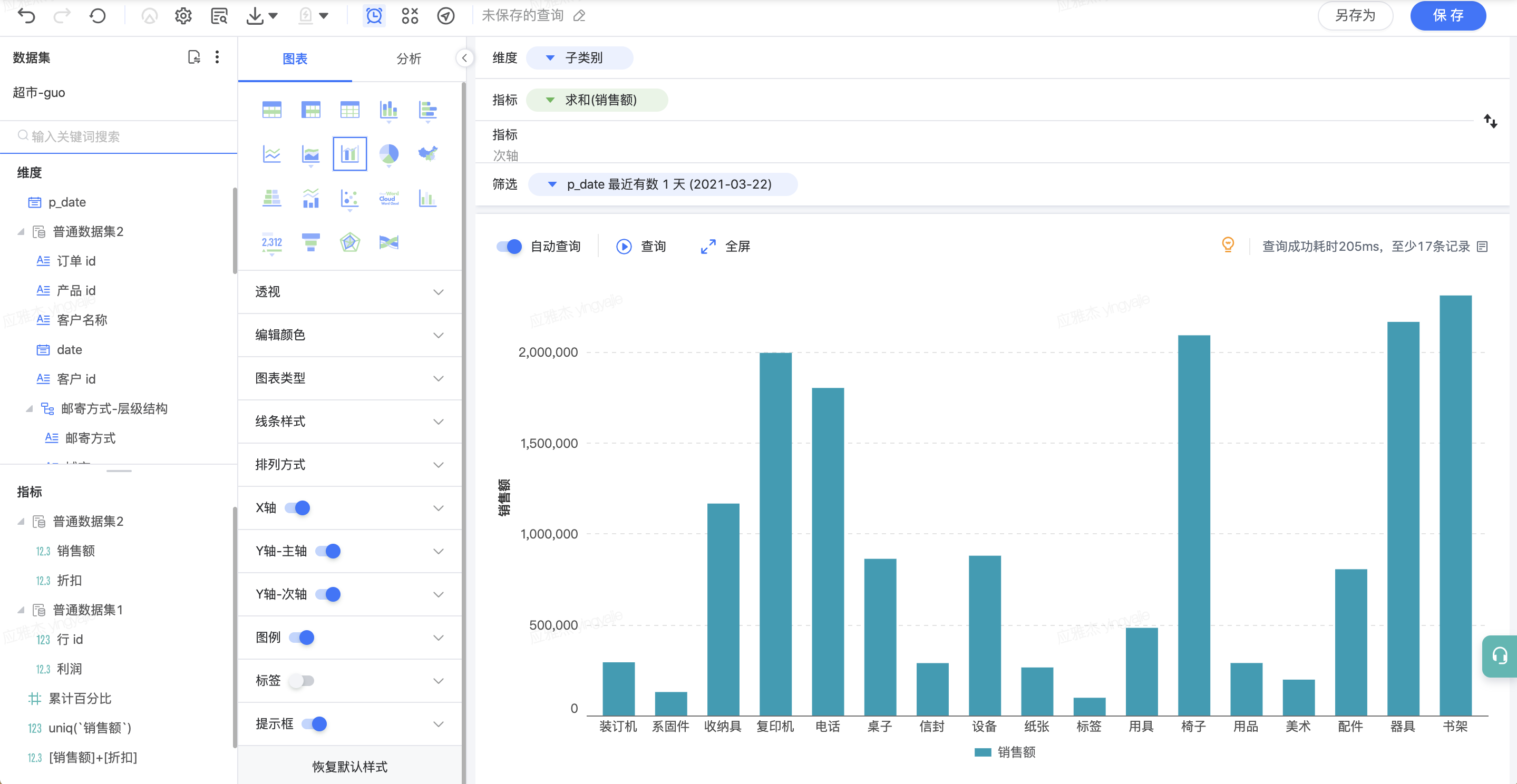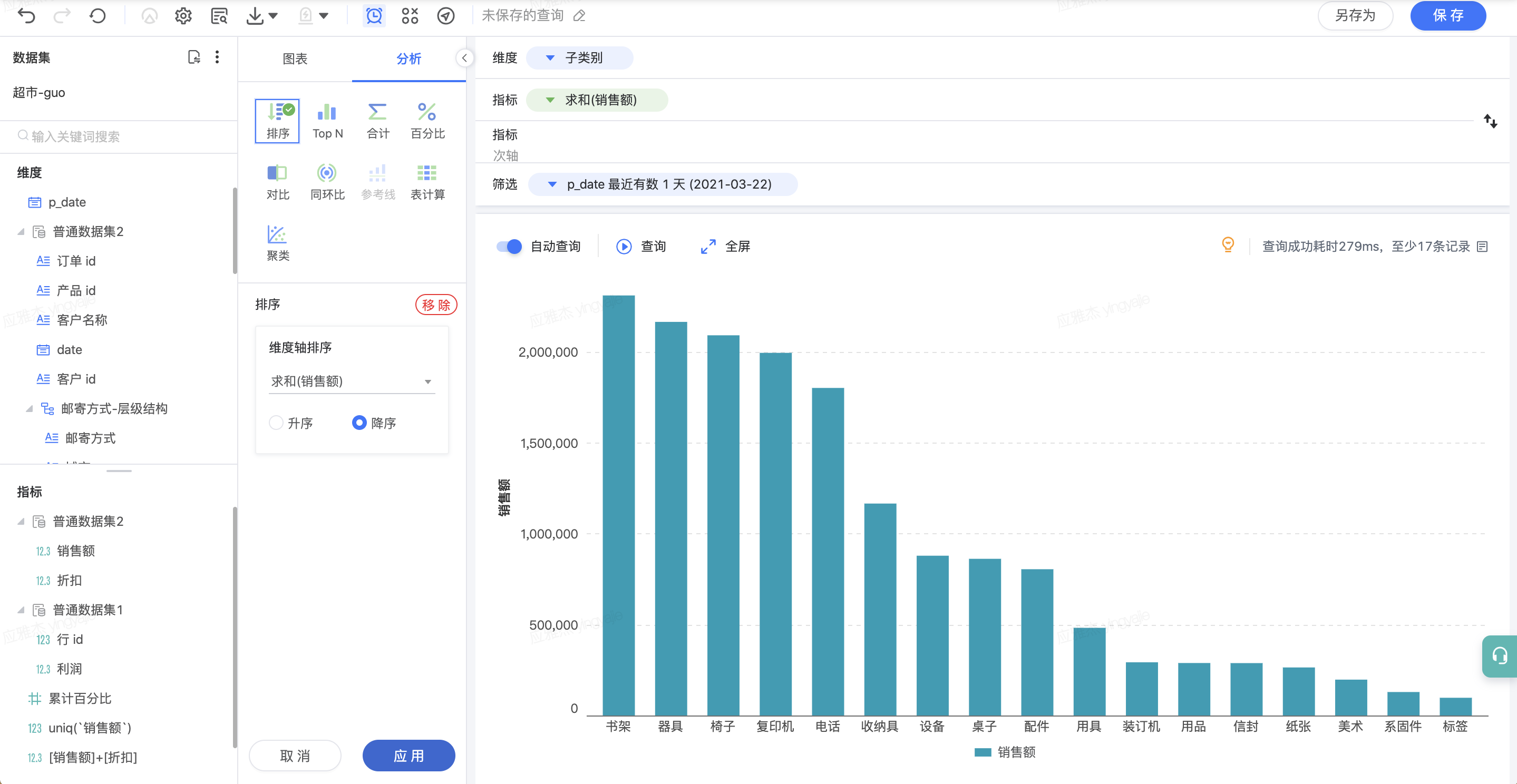running_sum(sum([销售额])) along ([子类别])/total(sum([销售额])) along ([子类别])